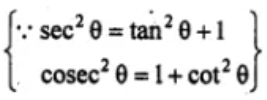Guru

# (i) cosec4 θ – cosec2 θ = cot4 θ + cot2 θ (ii) 2 sec2 θ – sec4 θ – 2 cosec2 θ + cosec4 θ = cot4 θ – tan4 θ.

• 1

This is an important question taken from the Book

Book- ML Aggarwal
Board- ICSE
Publication- Avichal
Chapter- Trigonometric Identities
Chapter number-18
We have to prove that equality holds true.

(i) cosec4 θ – cosec2 θ = cot4 θ + cot2 θ

(ii) 2 sec2 θ – sec4 θ– 2 cosec2 θ + cosec4 θ = cot4 θ – tan4 θ.

Class 10, chapter 18, trigonometric identities, ICSE board, ML aggarwal, question 20

Share

1. Solution:

(i) L.H.S. = cosec4 θ – cosec2 θ

= cosec2 θ (cosec2 θ – 1)

= cosec2 θ cot2 θ [cosec2 θ – 1 = cot2 θ]

= (cot2 θ + 1) cot2 θ

= cot4 θ + cot2 θ

= R.H.S.

(ii) L.H.S. = 2 sec2 θ – sec4 θ – 2 cosec2 θ + cosec4 θ

= 2 (tan2 θ + 1) – (tan2 θ + 1)2 – 2 (1 + cot2 θ) + (1 + cot2 θ)2= 2 tan2 θ + 2 – (tan4 θ + 2 tan2 θ + 1) – 2 – 2 cot2 θ + (1 + 2 cotθ + cot4 θ)

= 2 tan2 θ + 2 – tan4 θ – 2 tan2 θ – 1 – 2 – 2 cot2 θ + 1 + 2 cotθ + cot4 θ

= cot4 θ – tan4 θ = R.H.S.

• 1